# Tool for designing Butterworth and Chebyshev filters

Designing passive LC-filters typically involves looking up prototype filter component values in a table in reference books like Handbook of Filter Synthesis” by Zverev or Design of Microwave Filters, Impedance-Matching Networks, and Coupling Structures” by Matthaei et. al., then transforming the values to produce a filter with the desired impedance and cut-off frequency. This can be a bit tedious and error prone, so when I found out about the classic Matthaei book, which is downloadable via the link above, and that it contains the math required to calculate the prototype filter component value tables, I set out to create a spreadsheet to help with the filter design.

In addition to calculating the (previously) magical prototype tables for Butterworth and Chebyshev (with user-specified pass-band ripple) filters, the spreadsheet also performs the frequency and impedance transformation for filters of orders from 1 to 10.

To make the design process even quicker and better, I added a feature to create LTSpice schematics of the selected filter so that the filter properties can be simulated (and perhaps manually adapted to standard component values and to include parasitics) using LTSpice. I used the SI prefix formatting function I wrote about in the previous blog post to write out the component values in a pretty manner.

The usage of the spreadsheet should be fairly self-explanatory, but there are also usage instructions on the first tab. Basically, the user should fill out the values in yellow cells and leave the rest alone. I did not lock any cells, since I often get annoyed by spreadsheets with locked cells and I encourage others to modify and improve it.

Here is a link to the Excel 2002 file:FilterSynthesis_v1.0.xls

Make sure that macros are enabled if you want to use the LTSpice export features.

### Here are some screenshots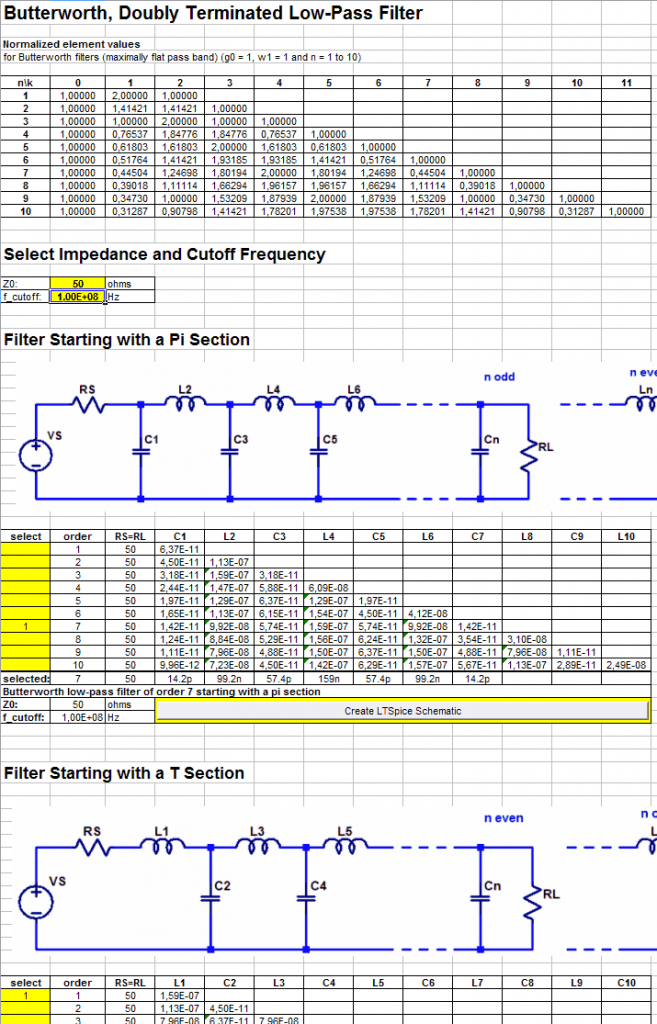Butterworth tab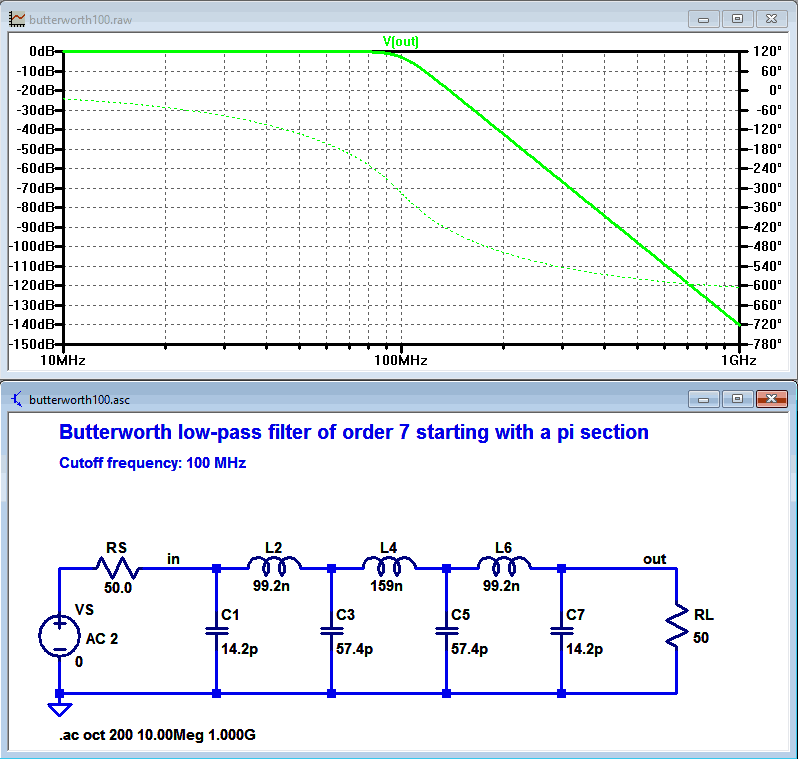LTSpice simulation of a Butterworth schematic generated by the spreadsheet.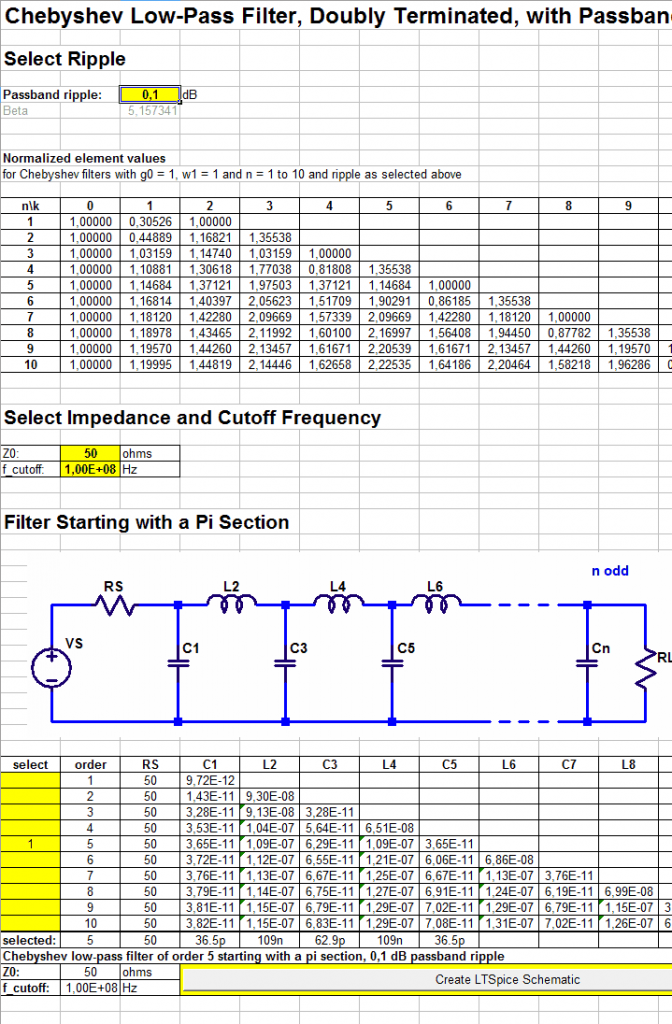Chebyshev tab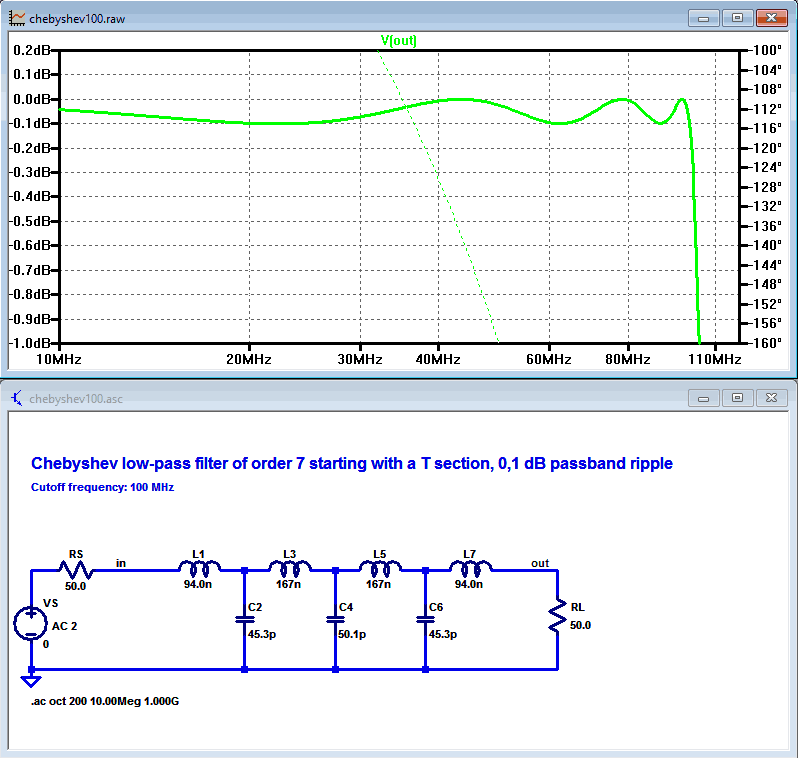LTSpice simulation of a Chebyshev schematic generated by the spreadsheet.

# Excel VBA functions for formatting numbers using SI prefixes (and back)

Excel does not have any built-in way of formatting numbers such that the numbers are displayed in a neatly readable way using SI prefixes, like m for milli (10^-3), k for kilo (10^3), µ for micro (10^-6) etc. Here I provide a VBA function I wrote to fill this need. In addition, I added support for using the prefix notation of Spice circuit simulators where both m and M are interpreted as milli and Meg has to be used to signify mega. Also, u has to be used instead of µ to indicate micro in Spice.

As icing on the cake, I also wrote a function to convert the resulting strings back to numbers.

The functions are called FormatPrefix and ValPrefix. Source code with detailed descriptions of the input arguments are shown further down in this post.

Below is a link to an Excel document with the functions built into a VBA module and a worksheet with an example table of values fed to the function to display its output for a large variation of input arguments: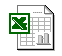ExcelPrefixFormattingFunction.xls

Here is a screenshot demonstrating the formatting: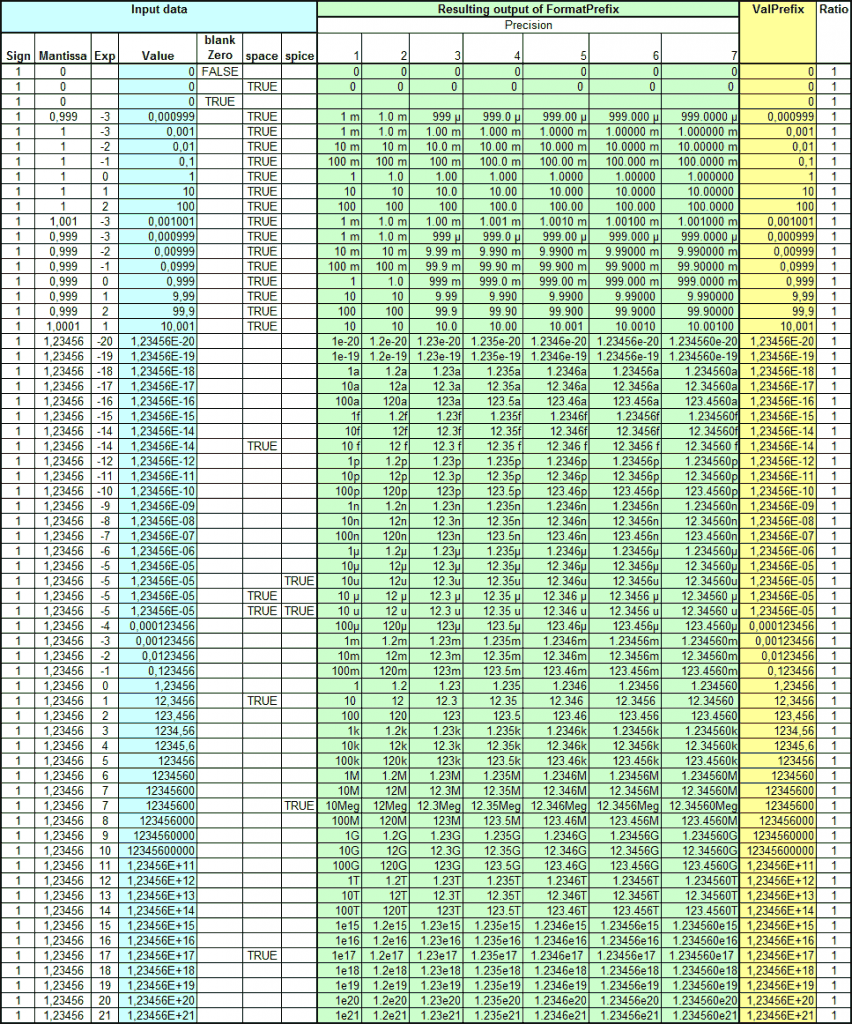Excel table showing the output of FormatPrefix for a large set of input arguments.

The source code is shown below:

```Public Function FormatPrefix(value As Double, _
Optional precision As Integer = 3, _
Optional blankZero As Boolean = False, _
Optional space As Boolean = False, _
Optional spice As Boolean = False) As String
' Convert a number to a string, usually with an SI prefix at the end.
'
' Arguments:
' value is the value to be converted.
' Optional precision argument determines number of significant digits to be printed (default 3).
' Optional blankZero argument determines if zero should return the empty string (default false).
' Optional space argument determines if a space should be inserted after the number but before
'  a possible prefix (default false).
' Optional spice argument determines if Spice prefixes should be used instead of metric ones
'  (default false). The only differences are Meg vs M and u vs µ.
' Values above or equal to 1e15 or below 1e-18 are printed using scientific notation without
' prefix.
' The decimal indicator is always a point, even if the locale says it should be a comma.
'
' Written by Per Magnusson, Axotron, 2016-02-26
' This code is public domain and comes without any warranty. Enjoy!

' Handle the value 0
If value = 0 Then
If blankZero Then
FormatPrefix = ""
Else
If space Then
FormatPrefix = "0 "
Else
FormatPrefix = "0"
End If
End If
Exit Function
End If

' Handle negative numbers
sign = 1
If value < 0 Then         sign = -1 ' store the sign         value = -value ' make value positive until later     End If          ' Calculate exponent and mantissa     exponent = Int(Log(value) / Log(10#))     mantissa = Round(value / (10 ^ exponent), precision - 1)     If mantissa >= 10 Then
' Handle the case where the mantissa is rounded up to 10
exponent = exponent + 1
mantissa = mantissa / 10
End If
exponent3 = 3 * Int(exponent / 3) 'exponent rounded down to nearest multiple of 3

' Find the right prefix
If exponent >= 15 Then
' Too big to represent with the supported prefixes, use scientific notation
prefix = "sci"
ElseIf exponent >= 12 Then
prefix = "T"
ElseIf exponent >= 9 Then
prefix = "G"
ElseIf exponent >= 6 Then
If spice Then
prefix = "Meg"
Else
prefix = "M"
End If
ElseIf exponent >= 3 Then
prefix = "k"
ElseIf exponent >= 0 Then
prefix = ""
ElseIf exponent >= -3 Then
prefix = "m"
ElseIf exponent >= -6 Then
If spice Then
prefix = "u"
Else
prefix = "µ"
End If
ElseIf exponent >= -9 Then
prefix = "n"
ElseIf exponent >= -12 Then
prefix = "p"
ElseIf exponent >= -15 Then
prefix = "f"
ElseIf exponent >= -18 Then
prefix = "a"
Else
' Too small to represent with the supported prefixes, use scientific notation
prefix = "sci"
End If

If prefix = "sci" Then
' Use scientific notation
formatStr = "0"
If precision > 1 Then
formatStr = formatStr & "."
For ii = 1 To precision - 1
formatStr = formatStr & "0"
Next
End If
formatStr = formatStr & "e-0"
prefix = ""
value = sign * mantissa * 10 ^ exponent
Else
decimals = precision - (exponent - exponent3) - 1
If decimals > 0 Then
formatStr = "0."
For ii = 1 To decimals
formatStr = formatStr & "0"
Next
Else
formatStr = "0"
End If
value = sign * mantissa * 10 ^ (exponent - exponent3)
End If
optSpace = ""
If space Then
optSpace = " "
End If
FormatPrefix = Replace(Format(value, formatStr) & optSpace & prefix, ",", ".")
'FormatPrefix = formatStr & optSpace & prefix
End Function

Public Function ValPrefix(str As String) As Double
' Convert a number formatted as a string using FormatPrefix() back to a number.
'
' Arguments:
' str is the string to be converted
' Error checking is very limited.
'
' Written by Per Magnusson, Axotron, 2016-02-26
' This code is public domain and comes without any warranty. Enjoy!

exponent = 0
prefix = ""
strlen = Len(str)

If StrComp(Right(str, 1), "A") >= 0 Then
' There is a letter at the end of the value
ii = 1
While StrComp(Mid(str, strlen - ii, 1), "A") >= 0
ii = ii + 1
Wend
prefix = Mid(str, strlen - ii + 1)
Select Case prefix
Case "T"
exponent = 12
Case "G"
exponent = 9
Case "M"
exponent = 6
Case "Meg"
exponent = 6
Case "k"
exponent = 3
Case "m"
exponent = -3
Case "u"
exponent = -6
Case "µ"
exponent = -6
Case "n"
exponent = -9
Case "p"
exponent = -12
Case "f"
exponent = -15
Case "a"
exponent = -18
Case Else
' Error, handle it somehow?
ValPrefix = "Prefix error"
Exit Function
End Select
End If
ValPrefix = Val(str) * 10 ^ exponent

End Function
```